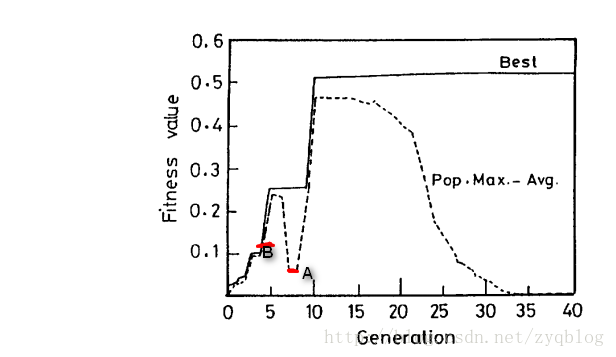动机

• 在找出包含最优解的范围之后，收敛到最佳效果的能力。（收敛能力）
• 探索解空间的新区域以寻找全局最优的能力。（寻优能力）

经过经验验证，适当的Pc值（0.5～1.0）和较小的Pm值（0.001~0.005）在传统遗传算法(SGA)实践中被普遍采用。而SGA存在着严重的缺点，它的Pc和Pm的值是固定的，不管是优良个体还是劣质个体都经过了相同概率的交叉和变异操作。这会引起两个很严重的问题：

• 相同的概率，这可以说是不公平，因为对于优良个体，我们应该减小交叉变异概率，使之能够尽量保存 ； 而对于劣质个体，我们应该增大交叉变异概率，使之能够尽可能的改变劣质的状况 。所以，一成不变的交叉变异概率影响了算法的效率。

• 相同的概率总不能很好的满足种群进化过程中的需要，比如在迭代初期，种群需要较高的交叉和变异概率，已达到快速寻找最优解的目的，而在收敛后期，种群需要较小的交叉和变异概率，以帮助种群在寻找完最优解后快速收敛。所以，一成不变的交叉变异概率影响了算法的效率。

自适应设计理念的诞生

AGA，旨在通过以不同的方式实现搜索和随机性之间的权衡，根据适合度值自适应地改变Pc和Pm的值 ，当群体倾向于停留在局部最优时（也就是群体适应度集中，多样性比较差时）Pc和Pm的值 增加，并且当群体在解空间中散布时（也就是群体适应度分散，多样性比较高时）减小。所以需要一个针对Pc和Pm的计算公式，让其符合动态变化。​ 我们注意上图中，（我们从整个种群的进化角度来看。）当GA收敛到适应度值为0.5的局部最优值时（全局最优解具有1.0的适应度值），Fmax-Favg减小了。Fmax-Favg作为GA检测收敛的标准，Pc和Pm值会根据Fmax-Favg的值进行变化。因为GA收敛到局部最优时，Pc和Pm的值必须增加，也就说，当Fmax-Favg减小的时候，Pc和Pm要增加（与Fmax-Favg的相反），也就说Fmax-Favg的值与Pc和Pm的值成反比。

$\begin{array}{}\text{(10)}& \left\{\begin{array}{r}\stackrel{.}{p}c={k}_{1}\frac{1}{fmax-favg},{k}_{1}\le 1.0\\ pm={k}_{2}\frac{1}{fmax-favg},{k}_{2}\le 1.0\end{array}\end{array}$

• Pc和Pm的值并不是依赖于特定个体的适应值，而是整个种群的平均和种群最大适应值 。因此优良个体和劣质个体都进行了相同水平的交叉和变异。 ，所以这对优良个体和劣质个体而言不公平，毕竟我们的初衷是想要保存优良个体，让交叉和变异小一点 ； 而改变劣质个体，让交叉和变异大一点。
• 当一个种群收敛到全局最优解的时候（甚至是局部最优解的时候），Pc和Pm增加，并且可能导致接近最优个体的损坏。种群可能永远不会收敛到全局最优解。即使我们能够阻止GA陷入局部最优解，但是GA的性能（根据收敛所需要的代数）肯定会恶化。

• 对于高适应度个体给予较低的Pc和Pm值，这有利于进行保存； 对于第适应度的个体给予高的Pc和Pm，这有利于劣质个体的改变。
• Pm的值不仅仅取决于Fmax-Favg,还取决于个体的适应值F。 F越接近与Fmax，Pm的值应该越小，即 Pm应该直接等于(Fmax-F )，相似的，Pc的值应该也直接等于(Fmax-F ’ ) （F‘ 是两个要交叉个体中适应度较大的那个适应度值）。

$\begin{array}{}\text{(11)}& \left\{\begin{array}{r}\stackrel{.}{p}c={k}_{1}\frac{fmax-{f}^{\prime }}{fmax-favg},{k}_{1}\le 1.0\\ pm={k}_{2}\frac{fmax-f}{fmax-favg},{k}_{2}\le 1.0\end{array}\end{array}$

$\begin{array}{}\text{(12)}& \left\{\begin{array}{r}\stackrel{.}{p}c={k}_{3},{f}^{\prime }\le favg\\ pm={k}_{4},f\le favg\\ {k}_{3}、{k}_{4}\le 1.0\end{array}\end{array}$

$\begin{array}{}\text{(13)}& pc=\left\{\begin{array}{r}{\stackrel{.}{k}}_{1}\frac{fmax-{f}^{\prime }}{fmax-favg},{f}^{\prime }\ge favg\\ {k}_{3},{f}^{\prime }\le favg\end{array}\end{array}$

$\begin{array}{}\text{(5)}& pm=\left\{\begin{array}{r}{\stackrel{.}{k}}_{2}\frac{fmax-f}{fmax-favg},{f}^{\prime }\ge favg\\ {k}_{4},{f}^{\prime }\le favg\end{array}\end{array}$

knum${k}_{num}$$k_{num}$ 的值

​ 在上述中，我们看到了一个适应度最高的个体，它的Pc和Pm都是0 。这个最好的个体被不停的复制到下一代。与选择机制一起循环，这可能导致这个最好个体在种群中以指数的增长形式快速增长，这会引起过早收敛。为了克服这个问题，我们为AGA（自适应遗传算法）的每一个个体都引进了一个默认的突变概率值（0.005）。

​ 在现有文献中已经阐明了Pc应该取适当的值，最好为 0.5<Pc<1.0，Pm应该取小一点的值，最好为0.001<Pm<0.005，这是普通遗传算法（GAS）成功的必要措施。Pc的取值能够促进广泛的交叉，Pm的取值为了防止个体被破坏。然而，当Pc和Pm的值不变化时，这个方法是有效的和有意义的。

​ 我们的方法的目标之一就是为了防止GA陷入局部最优解。为了实现这一个目标，我们采用低于平均适应度的个体去搜索空间中查找包含最优解的范围。像这种个体，需要被完全打乱，并且处于这种目的，我们让k4的值为0.5 。因为Favg的一个适应值的个体也应该被完全打乱，所以我们让K2的值也取0.5 。

总结

• 从种群整体来看：

• 从某一代种群内部各个个体来看：

• 参数问题选择：